# HBSE 7th Class Science Solutions Chapter 13 Motion and Time

Haryana State Board HBSE 7th Class Science Solutions Chapter 13 Motion and Time Textbook Exercise Questions and Answers.

## Haryana Board 7th Class Science Solutions Chapter 13 Motion and Time

HBSE 7th Class Science Motion and Time Textbook Questions and Answers

Question 1.
Classify the following as motion along a straight line, circular or oscillatory motion :
(i) Motion of your hands while running.
(ii) Motion of a horse pulling a cart on a straight road.
(iii) Motion of a child in a merry go round.
(iv) Motion of a child on a see-saw.
(v) Motion of the hammer of an electric bed.
(vi) Motion of a train on a straight bridge.
(i) Oscillatory
(ii) along a straight, line
(iii) circular
(iv) oscillatory
(v) oscillatory
(vi) along a straight line.

Question 2.
Which of the following is not correct?
(i) The basic unit of time is second.
(ii) Every object moves with a constant speed.
(iii) Distance between two cities are measured in kilometres.
(iv) The time period of a given pendulum is not constant.
(v) The speed of a train is expressed in m/h.
(ii), (iv) (v).

Question 3.
A simple pendulum takes 32 s to complete 20 oscillations. What is the time period of the pendulum?
Time period of a pendulum is time taken to complete 1 oscillation
Time taken to complete = 32 s.
20 oscillations
Time taken to complete = $$\frac { 32 }{ 20 }$$s.
1 oscillation
= 1.6 s.
∴ Time period of pendulum is 1.6 seconds.Question 4.
The distance between two stations is 240 km. A train takes 4 hours to cover this distance. Calculate the speed of the train.
Distance = 240 km.
Time taken = 4 hr.
Speed = $$\frac { Distance covered }{time taken }$$
= $$\frac { 240 }{ 4 }$$ $$\frac { km }{ h }$$
= 60 km/h.
Speed of the train is 60 km/h.

Question 5.
The odometer of a car reads 57321.0 km when the clock shows the time 08:30 AM. What is the distance moved by the car, if at 08:50 AM, the odometer reading has changed to 57336.0 km? Calculate the speed of the car in km/min during this time. Express the speed in km/ h also.
Distance = 57336.0 km – 57321 km = 15 Km
Time = 8.50 Am. – 8.30 Aim. = 20 min
Speed = $$\frac { Distance }{ time }$$ = $$\frac { 15 }{ 20 }$$ km/m
= 0.75 km/m. = 45 km/h

Question 6.
Salma takes 15 minutes from her house to reach her school on a bicycle. If the bicycle has a speed of 2 m/s. Calculate the distance between her house and’the school.
Time taken = 15 min
Speed = 2 m/s.
Distance = Speed x time
= 15 x 2
= 30 m.
So, distance between Salma’s School and her house is 30 metre.

Question 7.
Show the shape of the distance-time graph for the motion in the following cases :
(i) A car moving with a constant speed.
(ii) A car parked on a side road.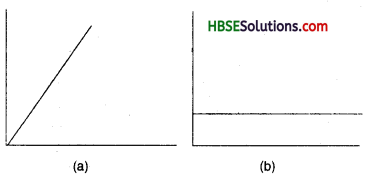Question 8.
Which of the following relations is correct?
(i) Speed = Distance x Time
(ii) Speed = $$\frac { Distance }{ Time }$$
(iii) Speed = $$\frac { Time }{ Distance }$$
(iv) Speed = $$\frac { 1 }{ Distance x Time }$$
(ii) Speed = $$\frac { Distance }{ Time }$$ is correct.

Question 9.
The basic unit of speed is :
(i) km/min
(ii) m/min
(iii) km/h
(iv) m/s
(iv) m/sQuestion 10.
A car moves with a speed of 40 km/h for 15 minutes and then with a speed of 60 km/h for the next 15 minutes. The total distance covered by the car is :
(i) 100 km
(ii) 25 km
(iii) 15 km
(iv) 10 km
(ii) 25 km

Question 11.
Suppose, the two photographs shown in Fig. 13.1 and Fig. 13.2, had been taken at an interval of 10 seconds. If a distance of 100 metres is shown by 1 cm in these photographs. Calculate the speed of the blue car.
0.1 cm/s or 10 m/s.

Question 12.
Fig. 13.16 shows the distance-time graph for the motion of two vehicles A and B. Which one of them is moving faster?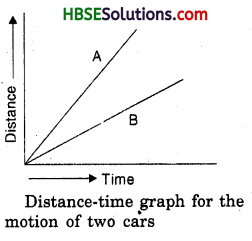‘A’ car is moving faster.

Question 13.
Which of the following distance-time graphs shows a truck moving with speed which is not constant?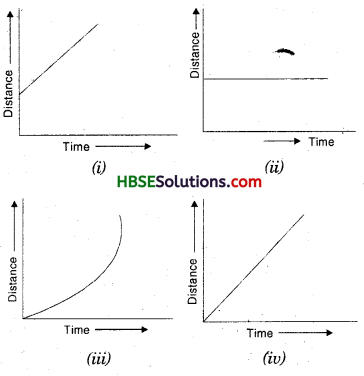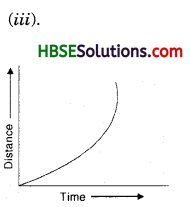Extend Learning – Activities and Projects

Question 1.
You can make your own sundial and use it to mark the time of the day at your place. First of all find the latitude of your city with the help of an atlas. Cut out a triangular piece of a cardboard such that its one angle is equal to the latitude of your place and the angle opposite to it is a right angle. Fix this piece, called gnomon, vertically along a diameter of a circular board a shown in Fig. 13.4. One way to fix the gnomon could be to make a groove along a diameter on the circular board.

Next, select an open space, which receives sunlight for most of the day, Mark a line on the ground along the North-South direction. Place the sundial in the sun as shown in Fig. 13.4 Mark the position of the tip of the shadow of the gnomon on the circular board as early in the day as possible, say 8:00 AM. Mark the position of the tip of the shadow every hour throughout the day. Draw lines to connect each point marked by you with the centre of the base of the gnomon as shown in Fig. 13.4. Extend the lines on the circular board up to its periphery. You can use „ this sundial to read the time of the day at your place. Remember that the gnomon should always be placed in the North- South direction as shown in Fig. 13.4.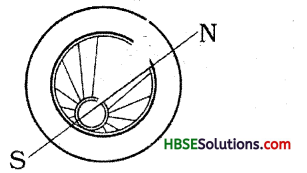Do it yourself.

Question 2.
Collect information about time-measuring devices that were used in the ancientitimes in different partrs of the world. Prepare a brief write up on each one of them. The write up may include the name of the device, the place of its origin, the period when it was used, the unit in which the time was measured by it and a drawing or a photograph of the device, if available.
Do it yourself

Question 3.
Make a model of a sand clock which can measure a time interval of 2 minutes (Fig. 13.5).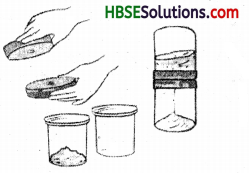Do it yourself

Question 4.
You can perform an interesting activity when you visit a park to ride a swing. You will require a watch. Make the swing oscillate without anyone sitting on it. Find its time period in the same way as you did for the pendulum. Make sure that there are no jerks in the motion of the swing. Ask one of your friends to sit on the swing. Push it once and let it swing naturally. Again measure its time period. Repeat the activity with different persons sitting on the swing. Compare the time period of the swing measured in different cases. What conclusions do you draw from this activity?
Do it yourself.

HBSE 7th Class Science Motion and Time Important Questions and Answers

Question 1.
What is motion?
Motion is the change in the position of a body with respect to time and its surroundings.

Question 2.
What is uniform motion?
When a body covers equal distance in equal intervals of time, the motion is called uniform motion.

Question 3.
What is speed?
Speed is the distance covered by a body in a unit time.Question 4.
Write one way in which people used to measure time in early days?
People used to measure time with the help of Sundials.

Question 5.
What is the use of stop-watch?
Stop-watch is used to measure exact time in case of athletic events as it can be stopped and started any moment.

Question 6.
What is a pendulum?
A pendulum is a small non-magnetic ball like body, suspended by a light string.

Question 7.
What is a uniform motion?
Motion of a body along a straight line covering equal distances in equal intervals of time is called a uniform motion.

Question 8.
What are the units of measuring speed?
Speed is measured in metre per second (m/s) or kilometre per hour (km/h).

Question 1.
Distinguish between uniform and non-uniform motion.
Uniform motion is the equal distances covered in equal intervals of time. It means body moves with a constant speed. Non-uniform motion is the unequal distances covered in equal intervals of time. It means the body moves with unconstant speed.

Question 2.
How can we make a pendulum of our own? What is the time period of a pendulum?
We can make a pendulum by susptending a metal ball with a cotton thread. The other end of the thread can be tied to some support. The time taken by a pendulum to complete its to and fro movement i.e. one oscillation is called the time period of the pendulum.

Question 3.
How can we determine the motion made by any body to be uniform or non-uniform with the help of a distance – time graph?
If the distance – time graph obtained shows a straight line, the motion of the body is said to be uniform and if we obtain a curved line on a distance time graph that means the body is undertaking non-uniform motion. Thus a distance time graph helps in determining the uniform or non-uniform speed of the body.

Question 4.
A train is running at a speed of 50 km/h. How long will it take to cover a distance of 250 km.?
Speed = 50 km/h
Distance = 250 km.
Time = ?
S = $$\frac { d }{ t }$$
50 = $$\frac { 250 }{ t }$$
∴ t = $$\frac { 250 }{ 50 }$$ hr = 5 hr.Question 5.
Shatabdi Express takes 6 hours to reach Lucknow, at a speed of 60 km/h. Find the distance it travels.
Time = 6 hr.
Speed = 60 km/h
Distance = Speed x time
= 60 x 6 = 360 km
∴ Shatabdi travels 360 km at a speed of 60 km/h in 6 hr.

Question 6.
A car travels a distance of 200 km at the speed of 50 km/h. Calculate the time taken to cover the distance.
Distance = 200 km
Speed = 50 km/h
Time = $$\frac { Distance }{ Speed }$$ = $$\frac { 200 }{ 50 }$$ = 4h
Car will take 4 h to cover the distance.

Question 1.
Given below is a table showing time taken by a car to travel various distances. What do you infer from this data?

 Time (S) Distance (M) 0 0 1 10 2 20 3 30 4 40 5 50

We see that the car covers the distance of first 10 m in 1 sec. It again covers the distance of 10 m in another time of 1 sec i.e. 2-1 = 1 sec. So, we find that the car covers every 10 m in each 1 sec. It means that the car is covering equal distance in equal interval of time. When a body covers equal distance in equal intervals of time, it is said to be in a uniform motion. So, this data shows that the car is moving with a constant speed.

Question 2.
Describe various methods used to measure time in earlier times?
There were no electronic watches in earlier times. Measuring time was a little bid difficult. The earliest method of measuring time was based on the position of the Sun. The Sundials were used for this purpose. Time was measured by the shadow casted by the changing position of the Sun from day to night. Chinese made a water clock 6000 years ago. Sand Clock was also used to measure time. Sand Clock was used by Romans. The time taken by sand to fall into the lower chamber from the upper chamber was considered to be the unit of measuring time. The discovery of pendulum helped in determining the exact time before the electronic watches were invented.

Question 3.
What is a pendulum? How does it help in determing time?
Pendulum is a simple device which shows periodic motion. A simple pendulum consists of a non-magnetic metal ball called bob. This bob is suspended with help of a string. The open end of the string is tied to a support. Bob of the pendulum is held at a side and released. It starts moving in a to and fro motion. This is called an oscillatory motion. The time taken by the pendulum to complete one oscillation is called time period. This time period is always same with a pendulum having same length. Time period changes with the change in the length of the string. The string of the pendulum is adjusted to the length, that it completes one oscillation in second and it keeps on moving the clock giving us time.

Motion and Time Class 7 HBSE Notes

• Motion is the change in the position of an object with respect to its surroundings.
• Motion can be of many types. It can be along a straight line, circular, oscillatory.
• Motion can be uniform or non-uniform motion.
• Uniform motion means equal distance covered in equal intervals of time. It means a constant motion.
• Non-uniform motion means unequal distance covered in equal intervals of time.
• Distance moved by an object in unit time is called its speed.
• Basic unit of measuring speed is (m/s) meter / second.
• Time is measured in respect to the periodic motions.
• Pendulum was the first device to measure time exactly.
• In earlier days time Was measured by various objects like sundials, sand clocks, water clocks, etc.
• With the discovery of pendulum, devices to measure exact time were invented.
• Now-a-days various devices like stop-watch and other electronic devices are used to measure time intervals smaller than even a second.
• Distance time graph gives us an idea about the motion of the object. Straight-line obtained on this graph depicts constant motion, while non-constant motion is depicted by various shapes on the graph.